GOLD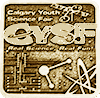#### Prognosing Idiopathic Pulmonary Fibrosis with Machine Learning

Statistical machine learning methods and neural networks were used to predict the future lung capacity of a patient of Idiopathic Pulmonary Fibrosis.

# Background

## Idiopathic Pulmonary Fibrosis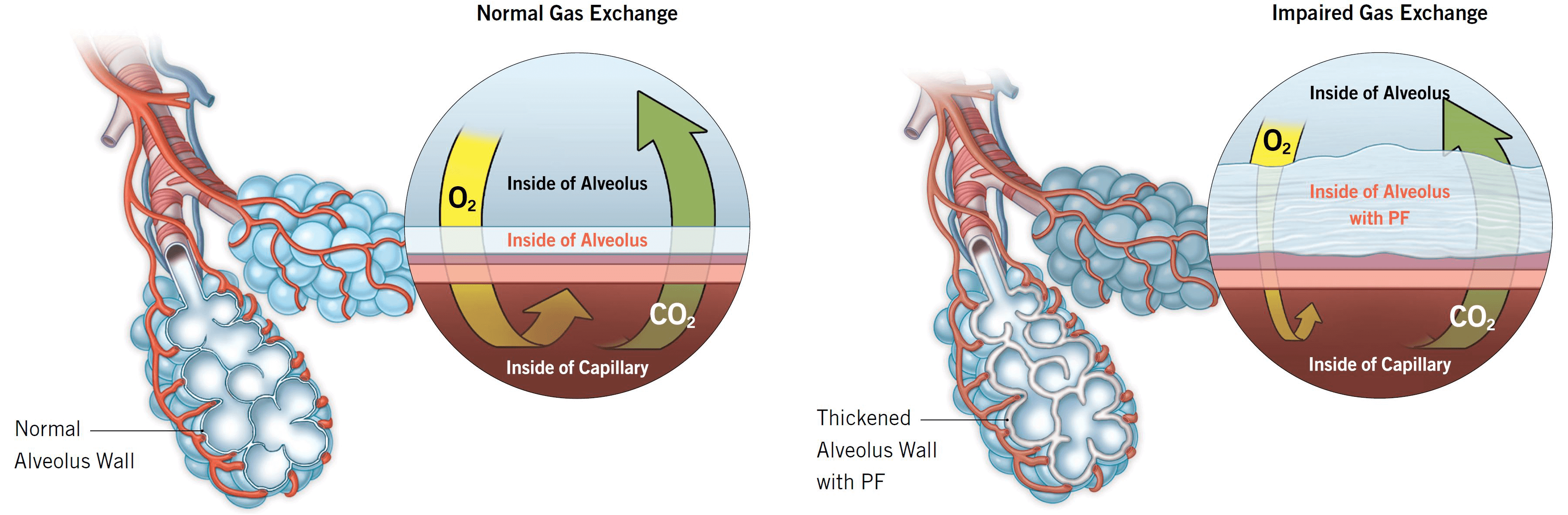Figure 1. Normal gas exchange vs Pulmonary Fibrosis caused impaired gas exchange. Image from pulmonaryfibrosis.org.

My project regards the disease Idiopathic Pulmonary Fibrosis or IPF. Here is some background information on the disease.

• A disease affecting the lung base and leads to lung function decline with no therapies available other than lung transplant (1, 2).
• Affects more than 5 out of every 100,000 individuals (1,3,4).
• It is age based and mainly affects older patients. The median age at diagnosis is 66 (4,5).
• Recent claims state that IPF is a result of abnormally activated alveolar epithelial
cells (5).
• Symptoms of IPF include the following (2).
• Shortness of breath
• Diffuse pulmonary infiltrates
• Varying levels of imflammation or fibrosis
• Sections of the lungs that are affected with IPF are characterized as follows (2).
• They alternate with unaffected areas
• There is a significant difference in cell age
• A honeycombing fibrosing pattern is created
• The severity can range from rapid health declination to a healthy stability and eventually death

There are several existing methods to prognose IPF, but none if them are perfect. Here are some of the existing prognosis methods.

• The cough scale questionnaire asks the patient about their level of fatigue after exercise (6-8)
• The 6 month 6 minute walk distance (6MWD) requires the effort of trained professionals to measure the effect of a 6 minute walk over 6 months (6, 9)
• A machine learning tool called CALIPER has been used with radiological changes to predict progression (10)
• Another method uses a CT scan based machine learning tool to categorize the severity of the disease with around 70% accuracy (11)

While these methods are not inaccurate, the existing methods have the following shortcomings.

• The measurements for prognosis take lots of time and professional effort
• The model categorizes the disease instead of predicting the future severity
• The prognosis method is not standardized

## Machine Learning Methods

Finding a good prognosis method for IPF is very important for the following reasons.

• It provides tangible metrics to recovery and progression of disease
• It enables patients to participate in clinical trials based on their IPF severity
• It gives an estimation of the patient's lifespan
• It helps patients determine which lifestyle changes to make to slow disease progression
• It gives patients and thier families time to cope and come to terms with the illness

Hence, finding a new prognosis method is important. Machine learning is a good solution for this problem because it does not require the active involvement of a medical professional and it has been used to prognose diseases in the past (12). similarily to how children are able to learn through example, machine learning focusses on getting computer to ba able to learn and make descisions. In specific, there is reason to believe that a machine learning prognosis would be successful and accurate because of the following reasons.

• Machine Learning has been used to prognose neck injury healing (14), prognose cancer (15), and even use CT scans to diagnose plant dieases (16).
• These show the versatility of machine learning and the validity of using a CT scan as a indicator of disease severity.
• It has also been proven that a CT scan imaging of the lungs contains sufficient insight to evaluate a prognosis (13).

# Problem

• This study aims to use a basline CT scan, and measurments of the forced vital capacity (FVC) of a patient's lungs over multiple doctor's checkups to predict the FVC of the patient in the future.
• The main questions of interest are as follows:
• What is the greatest accuracy a machine learning model can attain on predicting the future FVC?
• What model produces the greatest accuracy?
• Which model is of most use for doctors and medical professionals to use in their field?

### MethodFigure 2. A flowchart of the method employed for this project.

# Tools

• Python 3 (17)
• Tensorflow, Scikit Learn, and Pandas (18)
• Kaggle datasets and notebooks (19)

# Exploratory Data Analysis

## Data Features

The following are the features provided in the data.

• CT scans - DICOM formatted CT scans for each patient taken at week 0
• Patient ID - The unique ID to identify a patient
• Weeks - The number of weeks between the initial CT scan and the current measurements
• FVC - The patient’s lung capacity in mL
• Percent - The patient’s FVC as a percent of the FVC of similar patients
• Age - The patient’s age in years
• Sex - Male or Female
• Smoking Status - One of "never smoked", "ex-smoker", and "currently smokes"

## Patient Information

The dataset provided by the Open Source Image Consortium has information about 176 patients. Here are some graphs of the data.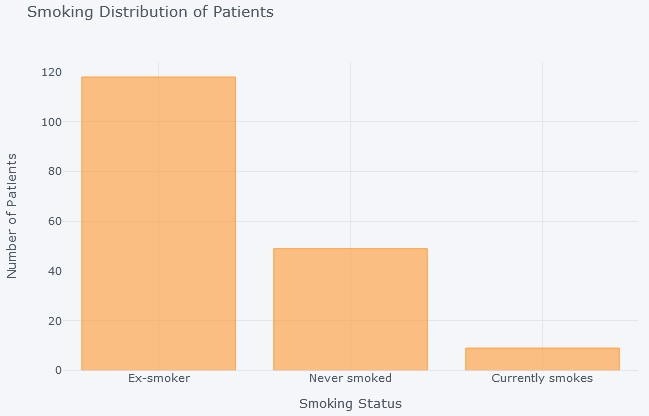Figure 3a. Patient Smoking DistributionFigure 3b. Patient Age DistributionFigure 3c. Patient Sex Distribution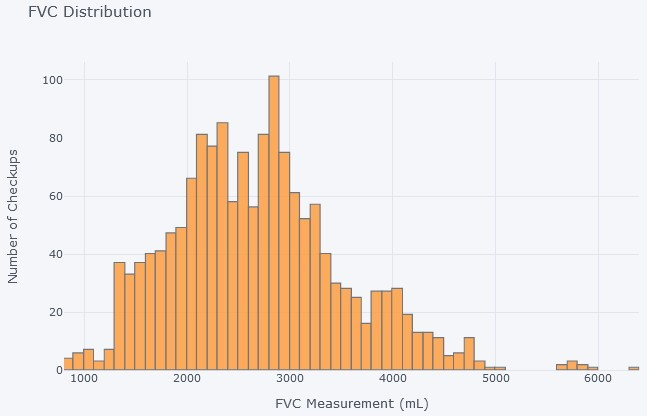Figure 3d. Patient FVC distribution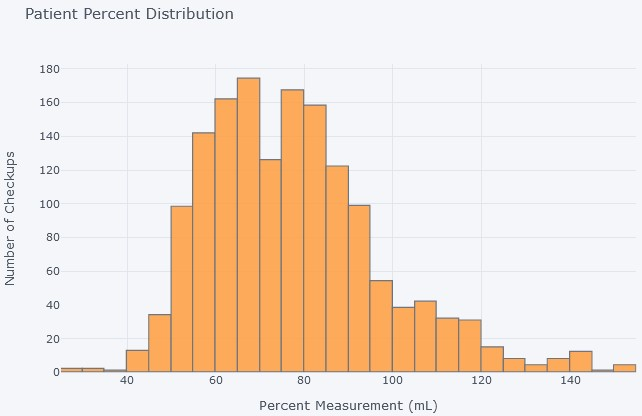Figure 3e. Patient Percent Ditribution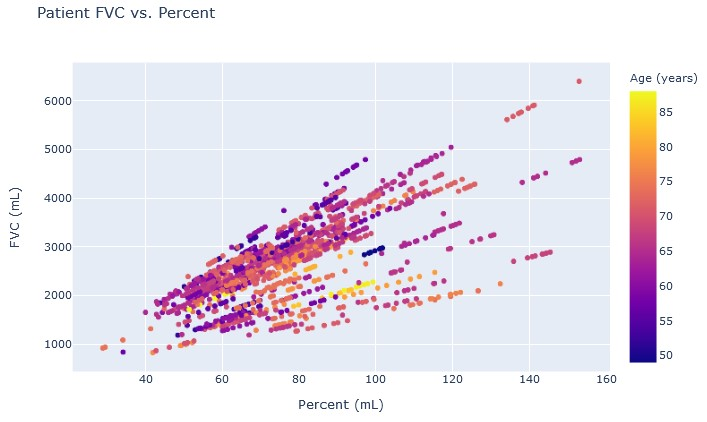Figure 3f. Correlation between FVC and Percent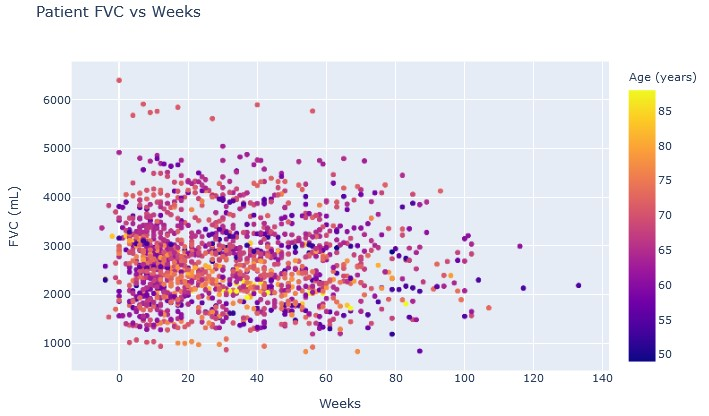Figure 3g. Patient FVC over time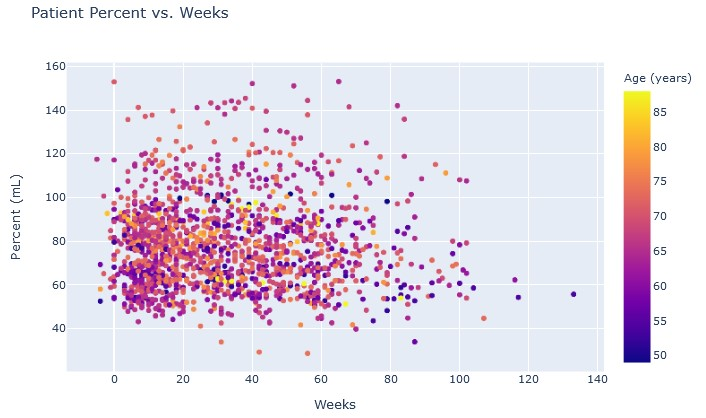Figure 3h. Patient Percent over time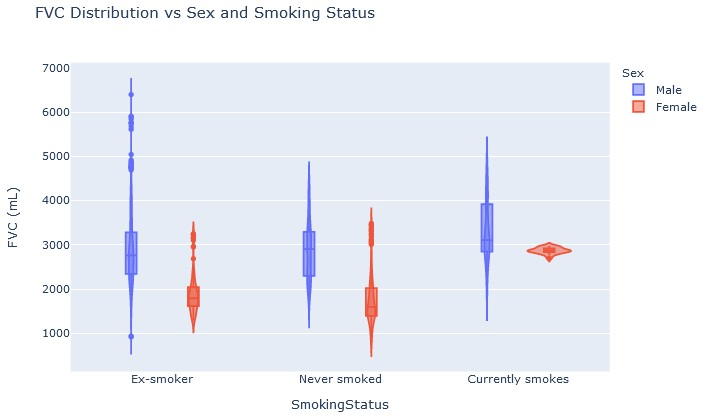Figure 3i. Patient FVC distribution vs Sex and Smoking StatusFigure 3j. Patient Percent distribution vs Sex and Smoking Status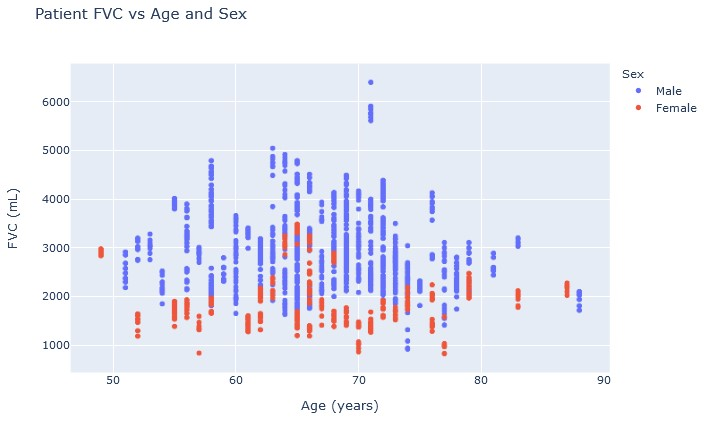Figure 3k. Patient FVC vs Age and Sex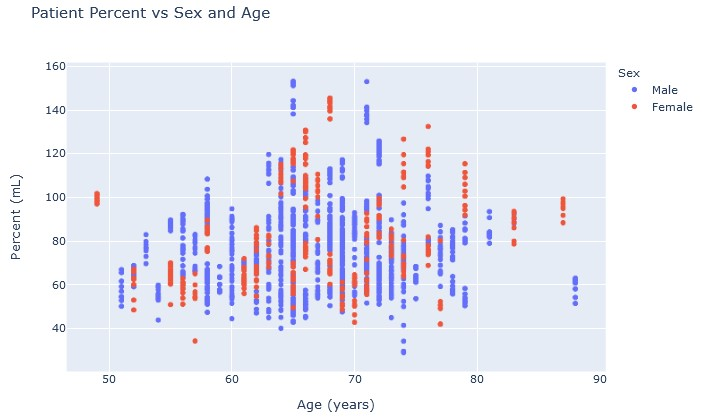Figure 3l. Patient Percent vs Sex and Age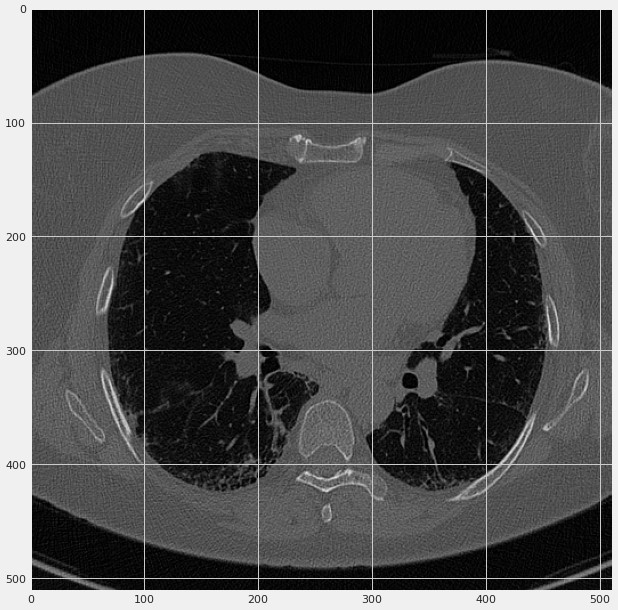Figure 3m. A slice of a patient's lung CT scan. Image from kaggle user Heroseo (20).

We can make the following obesrvations from the graphs.

• From figure 3b, we see the majority of patients are between 60 and 75 years old
• From figure 3c, we get that the majority of patients are male
• Figure 3f tells us that the percent feature is linearly correlated with the FVC of a patient
• Figures 3g and 3h tell us that while FVC decreases over time, the percent generally does not decrease as much over time
• Figures 3i and 3j seem to suggest that the FVC of female patients is more consistent, but this is likely due to the smaller sample size
• Figures 3k and 3l describe that while males naturally have a higher FVC, the percent feature takes that into account

The code for the data exploration can be found at https://drive.google.com/file/d/1eBvpJfHgEKJzh0KN9XOVlWC6B4aOKWZ2/view?usp=sharing.

# Models

## Linear Regression

The linear regreession method relies on the assumption that the FVC can be expressed as a linear combination of the features with some bias. In specific, the linear regression assumes the formula y = a1 * x1 + a2 * x2 + ... an * xn + b is true (where y is the FVC and x1 through xn are the imput features), then the model finds the values of the coefficients a1 through an and the bias b. Here is the procedure I followed when making the model.

1. Combined training and testing data together
2. Formatted combined data for use
3. Split the data back into training and testing sets
4. Created a linear regression model
5. Trained the model on the training data
6. Used the model to predict the FVC for the testing data
7. Calculated model accuracy

The code can be found at https://drive.google.com/file/d/1ySobiYd1-SmUdk9BG1pPdw5iWjLYH8us/view?usp=sharing.

## Simple Neural Network

The simple neural network is similar to the human brain. The model contains nodes connected to each other similar to the neurons in a brain, and the activation of the nodes depends on the nodes connected to the current node, the weights of the connections, and the node activation function. The following figure demonstrates the structure of a nueral network.Figure 4. Simple neural network example architecture. Image from medium.com (21).

Figure 4 shows that the input layer affects the values in the first hidden layer, which affects the values of the second hidden layer, which eventually affects the output layer value. Here is the procedure I used to make the model.

1. Combined, formated, and resplitted the data
2. Created multiple nueral networks with different architectures
3. Trained the models with the training data
4. Used the models to predict the FVC for the testing data
5. Chose the model which was most accurate on the testing data
6. Calculated model accuracy

## AutoEncoder

The base autoencoder was created by Kaggle user Welf Crozzo (22). The idea of an autoencoder is to use a neural network to strip an image into its elementary aspects, compressing it into a tabular form. The tabular data can then be used as input data for another model. This figure describes the process of encoding and decoding.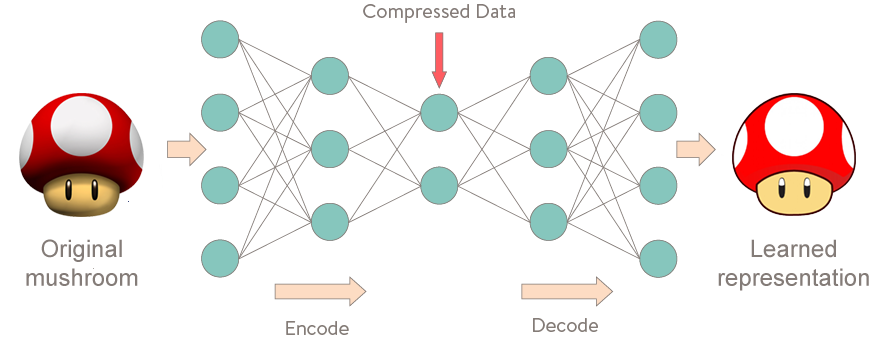Figure 5. Encoder and decoder system to compress images into tabular data, and reconstruct the image from the data. From medium.com (23).

In our case, we do not care about the decoder, but rather only the data produced by the encoder. Here are the steps I executed to make the model.

1. Loaded the AutoEncoder from Welf Crozzo's notebook
2. Combined the training and testing data
3. Used the encoder to generate an extra 2000 data features and format the data
4. Split the data into seperate training and testing sets
5. Built a linear regression and several simple neural networks with the data
6. Chose the best simple neural network
7. Used both methods to predict the FVC for the testing data
8. Calculated model accuracies

## Bayesian Learning

The bayesian learning method originates from Kaggle user Carlos Souza (24). I have made some modifications on the method. The bayesian model uses somethign called partial pooling. Each patient is fitted with their own individual linear curve, but all linear curves are related by a common distribution. The slope and y-intercept of the models are distributed according to a normal distribution, and the deviance of the model from the average model helps determine the confidence. The following diagram shows the how the predicted FVC is determined.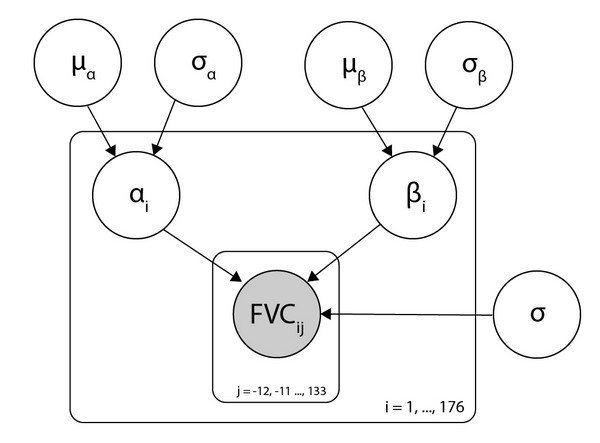Figure 6. Variables that determine FVC and confidence. Taken from (24).

Figure 6 shows us that each patient has their own linear curve (determined by alpha and beta), but that all curves are related (alpha and beta come from normal distributions). Below is the procedure to make the bayesian model.

1. Formatted data into a matrix completion task
2. Reduced the number of features
3. Created a partial pooling bayesian heirarchical model
4. Trained the model with the training data
5. Made predictions on the testing data, giving an accuracy based on the standard deviation
6. Calculated model accuracy

The code can be found at https://drive.google.com/file/d/1P6uJ2PiJaqNoShfMZiSPQZYGw1tz58Rh/view?usp=sharing.

## Multiple Quantile Regression

The multiple bayesian regression method is from Kaggle user Ulrich G. (25). The method uses convolutional neural networks and quantile regression to determine the model confidence. The quantile regression give the first and thrid quantiles which can be used to find a spread, and hence a measure of confidence. Here is a rough outline of how the model was made.

1. Formatted the data
2. Created a convolutional neural network from the tabular data
3. Trained the model with the training data
4. Used the quartile difference from the ground truth to get the model confidence
5. Used the model to predict the testing data FVC and confidence
6. Calculated model accuracy

The code can be found at https://drive.google.com/file/d/1tFUEscCDe9mDRH2HFLJoAfcxrpN8LdkM/view?usp=sharing.

## Linear Decay Theory

The linear decay method originates from Welf Crozzo's kaggle notebook (26). The linear decay method assumes that the FVC of the patient decays according to the formula FVC = a.quantile(0.75)*(week - weektest) + FVCtest, and that the confidence decays according to the formula Confidence = Percent + a.quantile(0.75)*|week - weektest|. A convolutional network (CNN) is then used to predict what the coefficient "a" is. Since a CNN is used, the CT scans can be analysed in this method. The following figure shows how a CNN interprets images.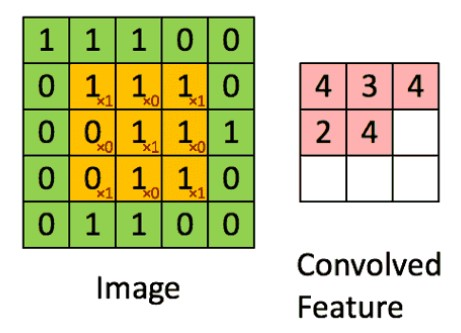Figure 7. CNN convoluting an image to be interpretted by the model. Image from towardsdatascience.com (27).

The figure above (figure 7) shows how images are interpretted by CNNs by becoming first being convoluted. The procedure to create a linear deacy model is below.

1. Formatted data
2. Created convolutional neural network to predict the coefficients of a linear FVC decay model
3. Trained the model
4. Used the linear decay equation to predict the test FVC and confidence
5. Calculated model accuracy

The code can be found at https://drive.google.com/file/d/11gi42dt2arhtXqEHJbgTFG9U33ZolkpK/view?usp=sharing.

## Lazy Predict

The final method, lazy predict, is not a single method, but rather a tool to compare multiple statistical methods at once to find out which will be the best to implement. Here is what I did to implement lazy predict.

1. Combined the training and testing sets, formatted the data, and reseperated the data
2. Used lazy predict to train several statistical models on the training data
3. Determine the statistical model with the lowest error

# Laplace Log Likelihood

The use of percent accuracy cannot be employed as the model is not given a categorization task, but rather a regression task. Using percent accuracy requires the model output to be discete, not continuous. For this reason, the use of the Laplace Log Likelihood (LLL) metric is employed to measure the model accuracy. The model's FVC prediciton, the true FVC, and the model's confidence are required to calculate the LLL. (Actually, confidence is a misnomer. A higher confidence score corresponds to a greater model uncertainty.)

A LLL closer to 0 represents a model which is more accurate, but the score 0 itself is unattainable for all practical purposes (due to the nature of the metric). An example of an outstanding score would be around -6.5.988

The worst score a model should get is -8.023. This score is attained as a result of always guesses the mean FVC, and always has a confidence of the standard deviation of the FVCs. Any model with a LLL lower than -8.023 is useless.

The following graph shows an example of how the model's confidence affects the metric. A confidence which is too high or too low is punished with a worse score. The local minimum describes the best metric obtainable when the predicted FVC is 2800mL, and the true FVC is 2500mL.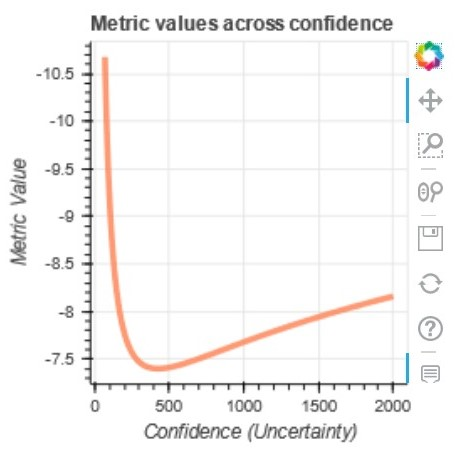Figure 8. Graph of LLL metric over model confidence for a true FVC of 2500mL and a predicted FVC of 2800mL. Taken from kaggle user Vopani (28).

# Model Analysis

## Overview

 Model Average LLL Advantages Disadvantages Linear Regression -6.813 - Does not overfit  - Is a statistical method - Assumes FVC is a linear function of features  - Does not use the baseline CT scan  - Gives a constant confidence value Simple Neural Network -6.868 - Does not assume how features affect FVC - The model gets stuck in local minimums while training, and doesn't properly optimize  - Does not used the baseline CT scan  - Gives a constant confidence value Linear Regression with Autoencoder Feautres -6.348 - Uses the baseline CT scan - Potential encoder overfitting  - Assumes FVC is a linear function of features  - Gives a constant confidence value Simple Neural Network with Autoencoder Features -11.623 - Does not assume how features affect FVC  - Uses the baseline CT scan - Potential encoder and model overfitting  - Many local minimums to get trapped in during training, making the model perform poorly  - Gives a constant confidence value Bayesian Method -6.641 - Gives a non-constant confidence value  - Is a statistical method - Assumes FVC is a linear function of features  - Does not use the baseline CT scan  - Slight overfitting Multiple Quantile Regression -6.884 - Gives a non-constant confidence value  - Doesn't assume how features affect FVC - Does not use the baseline CT scan  - Is prone to getting stuck in local minima, and not properly being optimized Linear Decay Theory -6.839 - Gives a non-constant confidence value  - Uses the baseline CT scan  - Is a statistical method - Assumes FVC decays linearly (as a function of time)

## Linear Regression

The following are the scores the linear regression recieved on the testing and training data.

Training data metric: -6.671
Testing
data private metric: -6.867
Testing data public metric: -6.902

The following scores are quite impressive, especially for a model as simple as linear regresssion. These are tough scores to beat. After training the model, the following graphs were produced.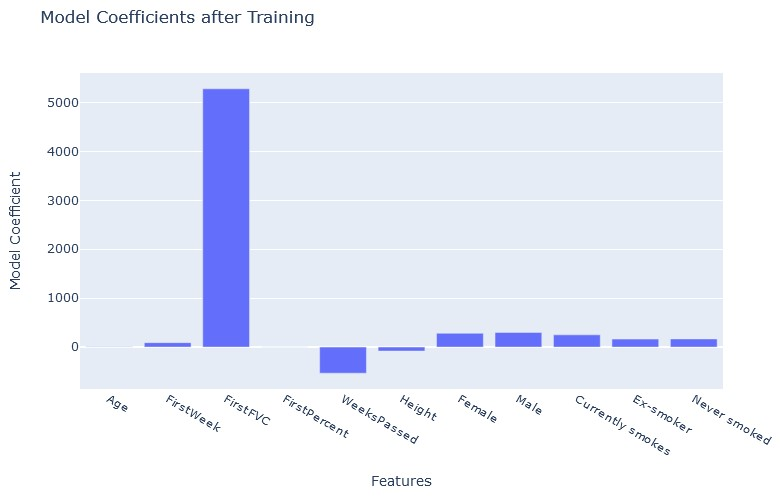Figure 9. Linear Regression Model Coefficient after Training

Figure 5a shows the relative importance of the model features. After the linear regression is trained, the feautures with high coefficients play a bigger role in the determination of the FVC. This graph shows us that the initial FVC recording and the number of weeks after the CT scan are the most important factors in determining the patient's final FVC.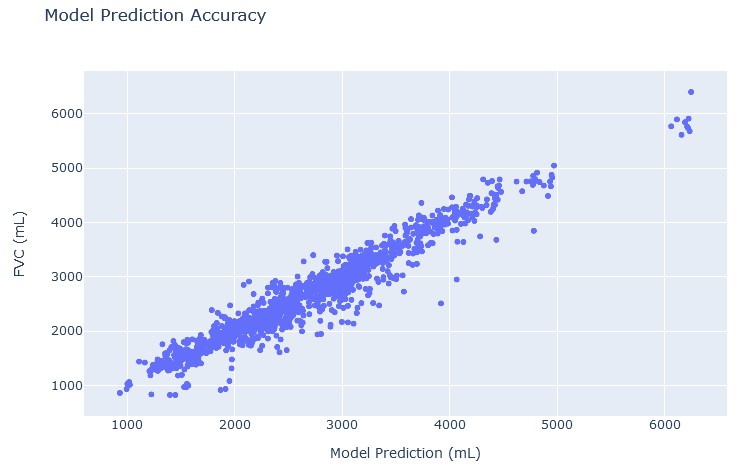Figure 10a. True FVC vs linear regression predicted FVC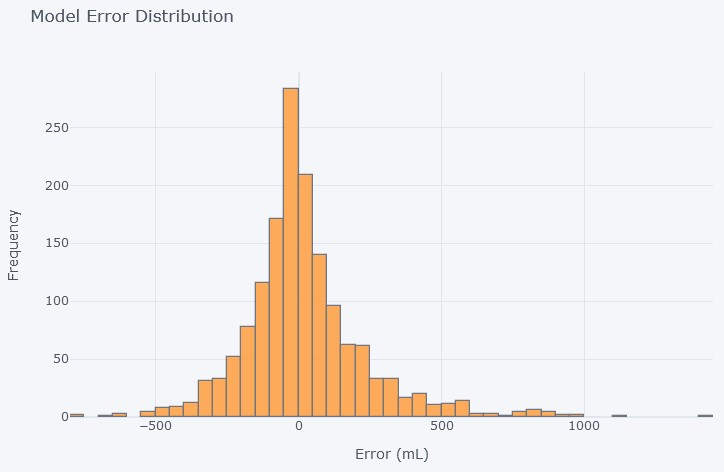Figure 10b. Linear regression model error

Figure 10a is a scatterplot with a roughly linear data trend. This means that the model indeed accurately predicts the true FVC since the data is almost the line y=x. Figure 10b shows the model error distribution. Since the distribution is unimodal and has a low spread, the model is relatively accurate.

## Simple Neural Network

The following are the scores the simple neural network recieved on the testing and training data.

Training data metric: -6.763
Testing
data private metric: -6.888
Testing data public metric: -6.953

We see that these scores are worse than those of the linear regression. This can likely be attributed to the fact that the model was stuck in a local minimum, and was unable to reach the optimal weights and biases. Perhaps a change in optimizer could remedy this problem. I have tried many optimizers, but found the optimizer ADAM to be most sucessful. Additionally, the original state of the weights and biases slightly affects the set of final weights and biases, something not in my control. I experimented with many model architectures to find the best architecture, which turned out to be a 11 x 64 x 64 x 1 model. The following graphs were produced after the model ran.Figure 11a. Model MSE over training epochs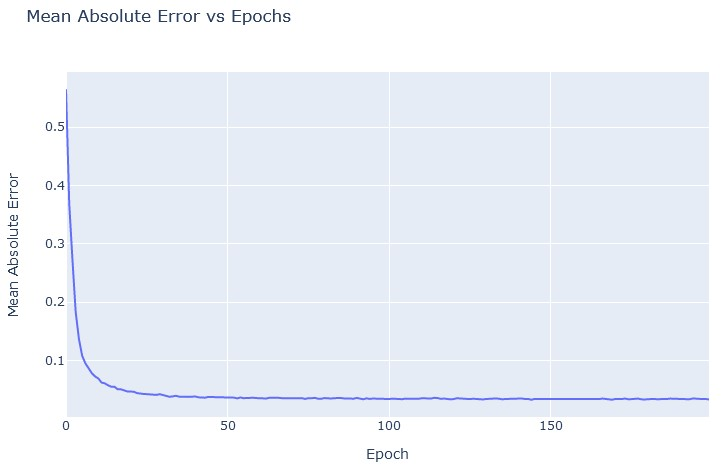Figure 11b. Model MAE over training epochsFigure 11c. Model loss over training epochs

Figures 11a, 11b, and 11c all demonstate that as the simple neural network trained, it quickly reached a local minimum. After reaching the local minimum at around 50 epochs, further training did not significantly improve the model performance.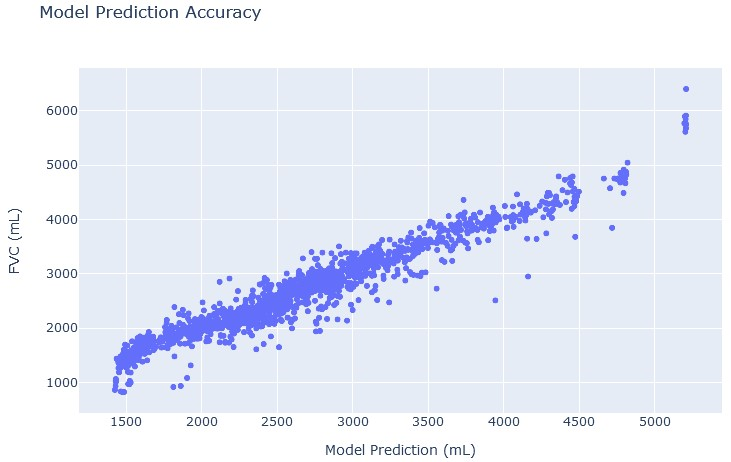Figure 12a. True FVC vs simple neural network predicted FVC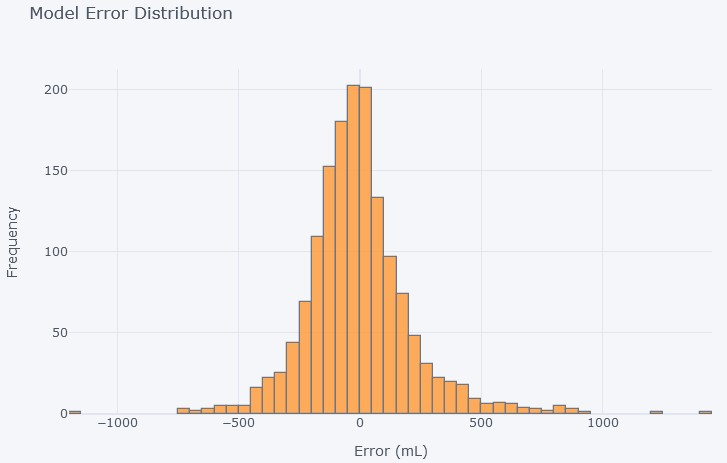Figure 12b. Simple neural network model error

Figures 12a and 12b show that the simple neural network did not model the true FVC as well as the linear regression, as figure 12a deviates from the line y=x, and figure 12b has a greater spread.

## Linear Regression with AutoEncoder generated features

The following is the score the linear regression with autoencoder model recieved on the testing data. The reason that there is no testing data score is because running both the linear regression and the autoencoder leads to a notebook timeout error on kaggle. This means I could not submit this method for scoring.

Training data metric: -6.348

This score far surpasses the scores of the linear regression and the simple neural network. One concern is that the linear regression is now overfitted, and this is likely true to some extent. This means that if this model were to be ran with testing data, then the LLL would be closer to -6.7 or -6.8. Here are two graphs of the model's performance.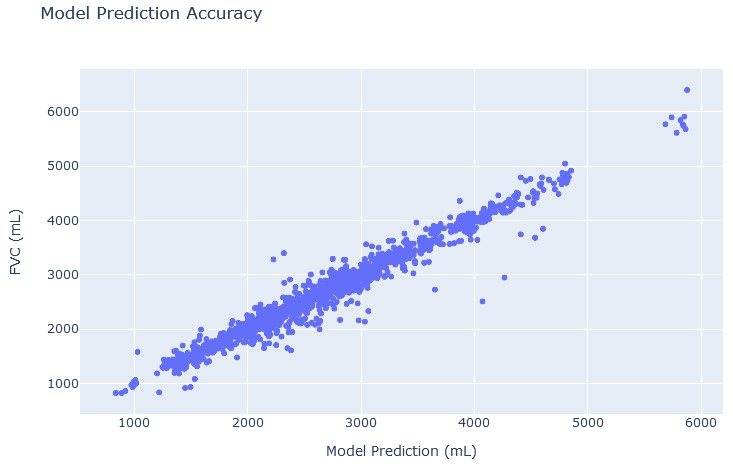Figure 13a. True FVC vs linear regression with autoencoder predicted FVC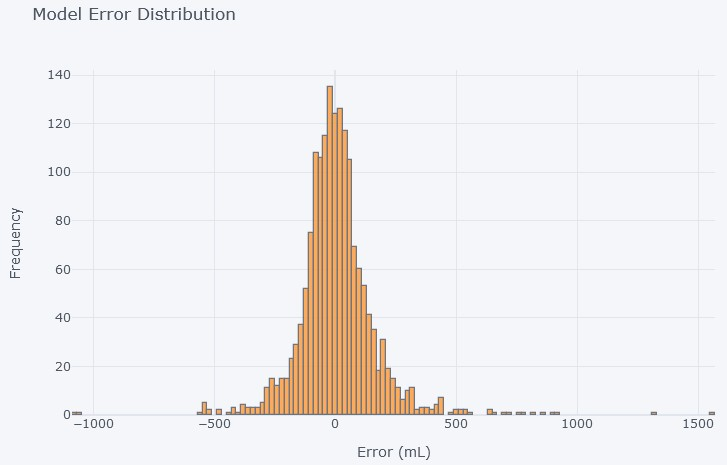Figure 13b. Linear regression with autoencoder model error

Figures 13a and 13b demonstrate that adding features to the linear regression with the help of the autoencoder did indeed improve the performance of the model.

## Simple Neural Network with AutoEncoder generated features

The following is the score the simple neural network with autoencoder model recieved on the testing data. Again, there is no testing data score for the same reason.

Training data metric: -11.623

It is interesting to note that this model actually performed worse than all the other models. I predict that this is because very few of the input features actually have a very significant role in the calculation of the FVC, and this makes the model very prone to getting caught in local minimums, and never reaching the optimal weights and biases. You would expect this model to perform at least as well as the simple neural network, but the number of input features and choice of optimizer did not lead to that result. Again, I experiemented with optimizers and architectures, but ended up using an RMSprop optimizer and a 1931 x 1831 x 1 architecture.Figure 14a. Model MSE over training epochs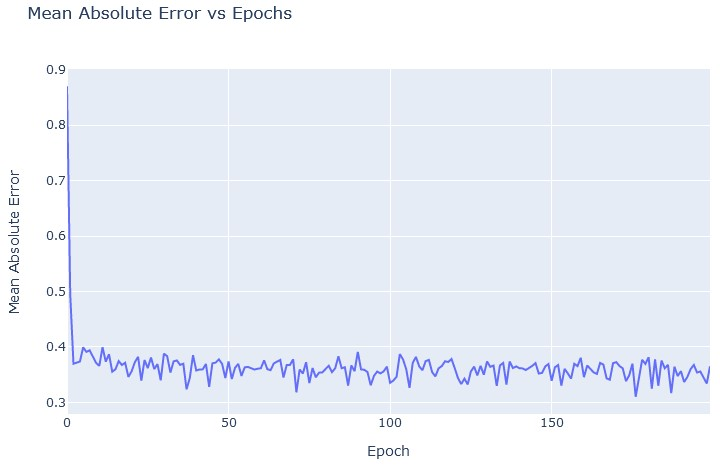Figure 14b. Model MAE over training epochs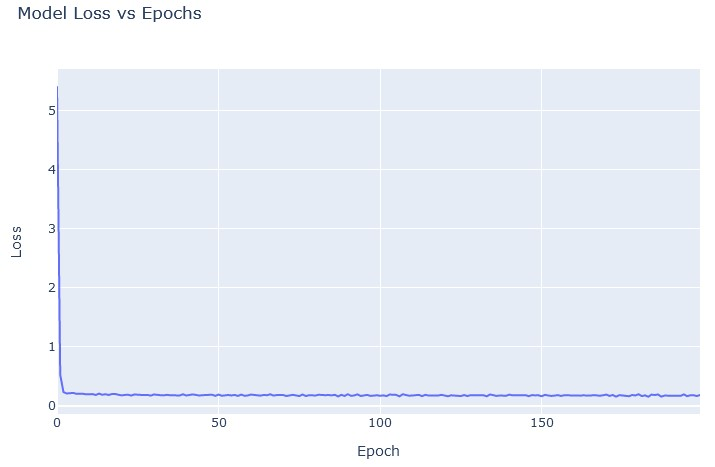Figure 14c. Model loss over training epochs

Figures 14a, 14b, and 14c all demonstrate that the model was still quite unpredictable at the end of the training sesion, and that it likely did not reach the global minimum.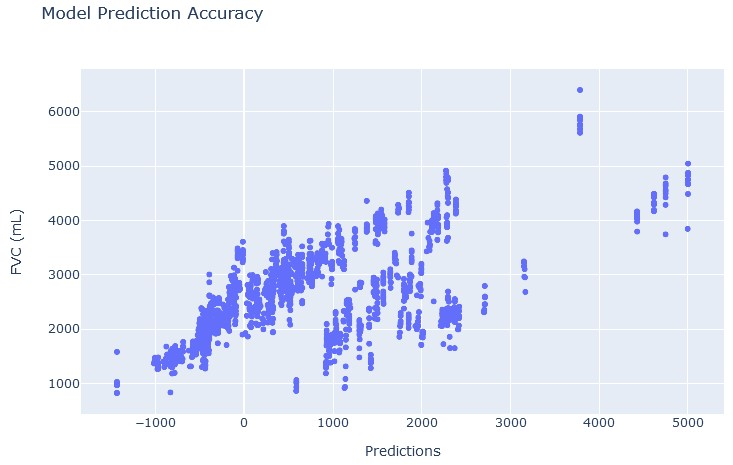Figure 15a. True FVC vs neural network with autoencoder predicted FVCFigure 15b. Simple neural network with autoencoder model error

figure 15a shows the poor accuracy the model had when predicting the true FVC. Figure 15b also shows the wide ranges of error of the model. The model was not even unimodal, and did not even have a mode of 0.

## Bayesian Model

The following are the scores the bayesian model recieved on the testing and training data.

Training data metric: -6.146
Testing
data private metric: -6.868
Testing data public metric: -6.909

These scores are comparable to the scores of the linear model, but the bayesian model did perform better on hte training data. This is due to overfitting. The nature of this model leaves a high likelihood of overfitting, and the difference in testing and training score difference supports the hypothesis of overfitting. Here are some graphs of the model after training.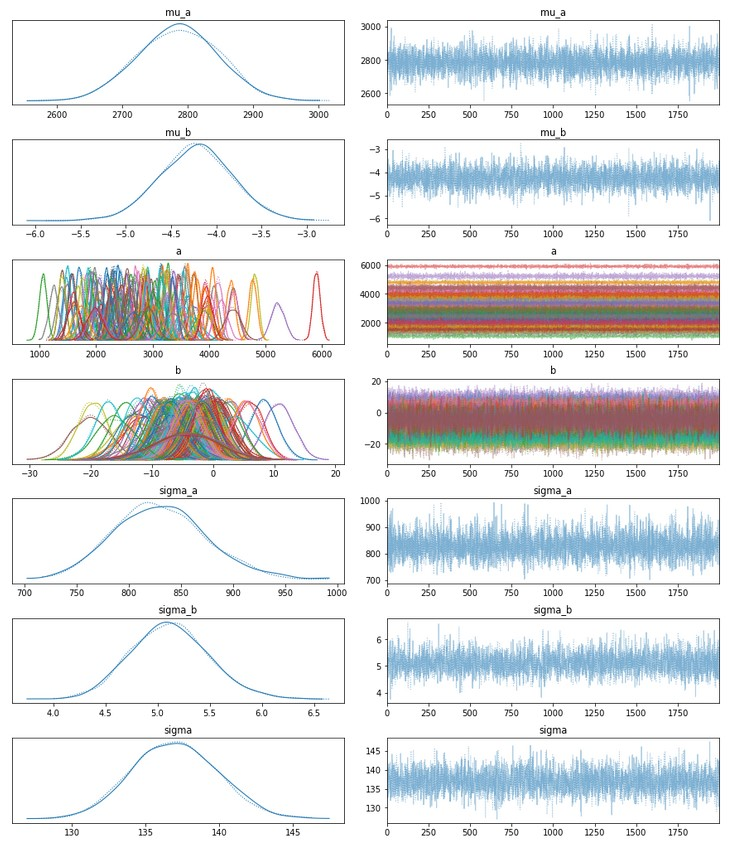Figure 16a. Bayesian model learned variables. From (24)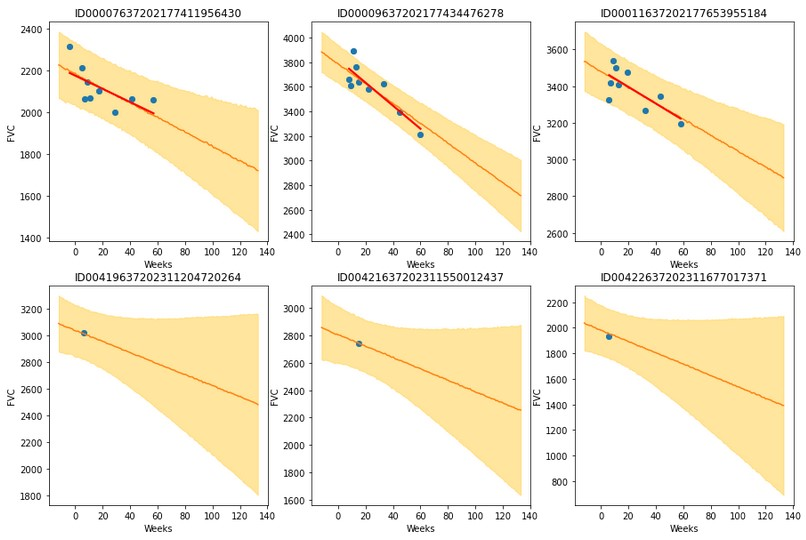Figure 16b. 6 patients whose individual linear curves were graphed. From (24)

Figure 16a shows us the learned distributions for each of the varibales used in the partial pooling method. We see the ditribution of the vairables is roughly normal as we would expect, and that there is indeed a variety in the ditributions for each a and b. This is to be expected. Figure 16b demonstrates how the model's confidence varies. The model's uncertainty (the yellow section) increases as predictions further in the future are made.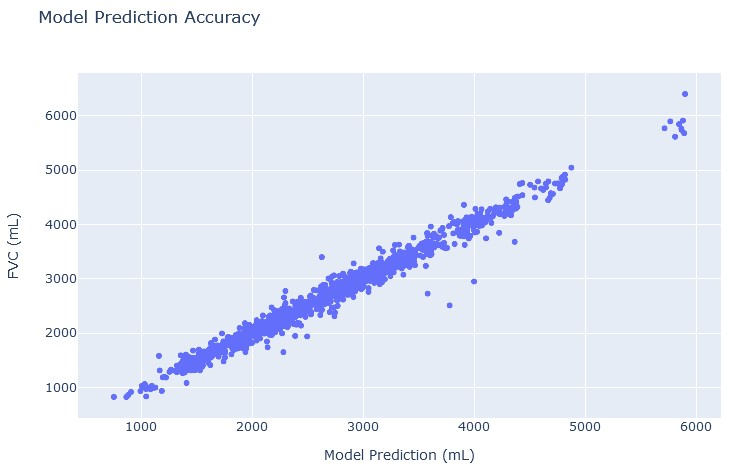Figure 17a. True FVC vs bayesian predicted FVC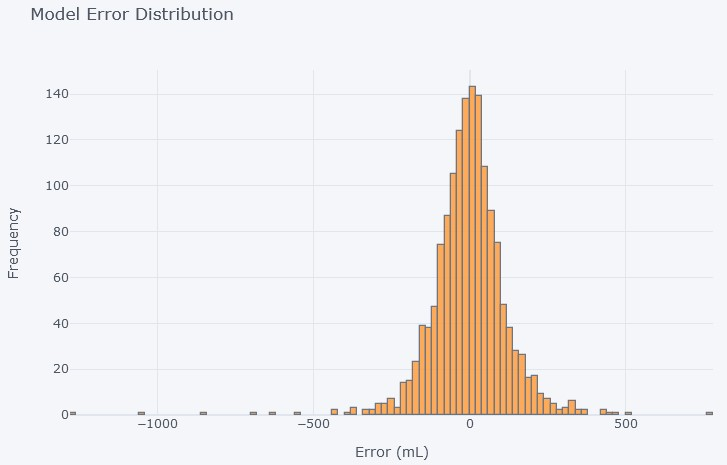Figure 17b. Bayesian model error distribution

Figure 17a very accurately resembles the line y=x, which means that the bayesian model has learnt the training data to a very high level of accuracy. Figure 17b reinforces this idea as the model error is unimodal, and has a low spread.

## Multiple Quantile Regression

The following are the scores the multiple quntile regression recieved on the testing and training data.

Testing data private metric: -6.922
Testing data public metric: -6.845

Although the model results are not as good as those for models such as linear regression, or the bayesian model, we see that the results are comparable to the simple neural network. On the other hand, though, this methods does provide a good and useful measure of confidence.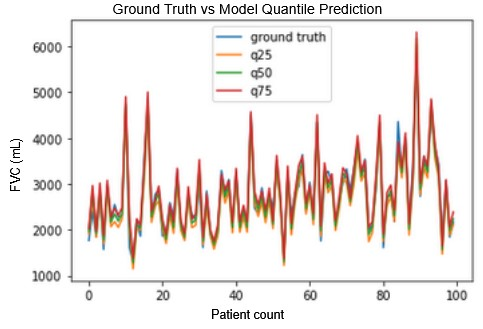Figure 18a. Model quantiles vs ground truth. From (25)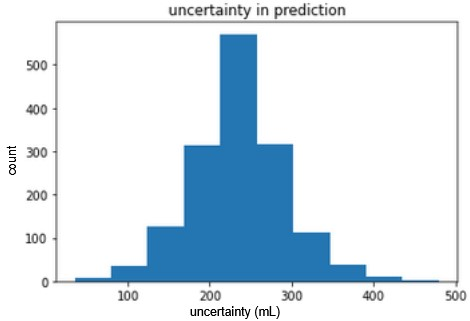Figure 18b. Model confidence (uncertainty) distribution. From (25)

Figure 18a demonstartes that the quntile regression models are relatively close to the gorund truth but they have a nonzero spread corresponding to the model confidence. Figure 18b shows the distribution of model uncertainty. The mode is around 250 mL which is around equal to the standard devation of the FVCs.

## Linear Decay Model

The following are the scores the linear decay model recieved on the testing and training data.

Training data metric: -6.723
Testing
data private metric: -6.877
Testing data public metric: -6.918

These scores are very good and can be compared to the scores of the multiple quantile regression method. While the scores are similar, we see that the private and public scores are almost swapped. This can be attributed to the fact that certain methods perform differently according to the data provided. Luckliy, the scores are very close to each other, indicating a low likelihood of overfitting.

## Lazy Predict

When running lazy predict, the models with the highest scores were the following.

• Random Forest Regression
• Extra Tree Regressor

These are the models that I should implement in the future if I have more time. They will likely prove to become good fits of the data.

# Comparison

After creating and running several methods to prognose IPF, the following model results were obtained.Figure 19a. Model Performance according to testing set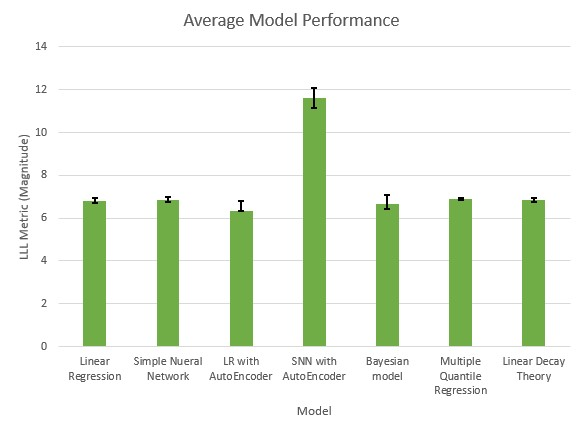Figure 19b. Average model performance with error intervals

The figure above (figure 19) shows the relative model scores with the LLL metric. A shorter bar correlates to a better performance. This graph demonstrates that the best models according to their LLL scores are the linear regression, linear regression with autoencoder, and bayesian models. We see from figure 19b that different figures have different error ranges, and including these error bars, it seems that the most consistent models are multiple quantile regression and linear decay theory.

# Conclusion

A number of machine learning models were created which prognose IPF. From the analysis section, we saw that the bayesian model, linear regression, and linear regression with autoencoder have the best LLL scores. Even though those are the models with the best scores, the models that are most reliable and useful in the medical field are the bayesian model, and the linear decay theory. These methods are prefered in medicine for the following reasons.

• They are statistical methods (so the way prediction occurs is well understood)
• They provide a non-constant measure of confidence which is useful to doctors

Overall, use of the linear decay method is most recommended. It not only has a very good LLL score, but it also has a potential to be of good use to doctors in prognosing IPF and does not overfit the training data like the bayesian model.

Overall, though, we can see that the simplest models with the fewest assumptions are often the most successful. Furthermore, this project depicts the importance of using statistical methods and providing a measure of confidence in medical fields.

# Significance

The results of this project allowed the accurate and successful prognosis of IPF. The use of the linear decay theory could do the following for patients suffering with IPF.

• Elimainate human bias in the prognosis by the doctor
• Give patients enough time to come to terms with their disease and look into what lifestyle changes they can make to slow the progression

Additionally, the lessons learnt from this project can be applied to many other diseases as well. Namely, the lesson of not overcomplicating the model can be applied to other projects. A similar conclusion has been made before in many other projects, and has been described by Occam's razor. Occam's razor states that when there are multiple competing hypothesis (the multiple models being compared), the hypothesis with the simplest assumption (the assumption that FVC is a linear function of features) is the best hypothesis.

# What's Next

To improve my project, I could do the following in the future.

• Implement the models based on the results of the lazy predict
• Create a web application which medical professionals can use to prognose IPF
• Use machine learning to segment the lungs and find which parts of the lungs are important in the severity of IPF

These could make the predictions more accurate and help medical professionals.

### Acknowledgement

A special thank you to Dr. Garcia Diaz and Dr. Christian Jacob for supervising my project and mentoring me.